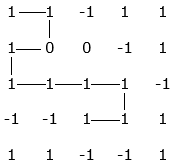# Collect maximum points in a matrix by satisfying given constraints

Given a M x N matrix where each cell can have value of 1, 0 or -1, where -1 denotes a unsafe cell, collect maximum number of ones starting from first cell and by visiting only safe cells (i.e. 0 or 1). We are allowed to go only left or down if the row is odd else, we can only go only right or down from current cell.

For example, consider below matrix shown on the left. The maximum value collected is 9 as marked belowThe idea is to use recursion. The problem has an optimal substructure. That means the problem can be broken down into smaller, simple “subproblems”, which can further be divided into yet simpler, smaller subproblems until the solution becomes trivial. For matrix M, the problem can be recursively defined as –

if (M[i][j] != -1)
path(i, j) = M[i][j] + | max(path(i, j – 1), path(i + 1, j))  (if i is odd)
| max(path(i, j + 1), path(i + 1, j))  (if i is even)
else
path(i, j) = 0

Here, path(i, j) calculates the maximum value that can be collected starting from cell (i, j).

Below is C++ and Java implementation of the idea:

## C++

Output:

Maximum value collected is 9

## Java

Output:

Maximum value collected is 9

The time complexity of above solution is exponential and auxiliary space used by the program is O(1).

The problem clearly exhibits overlapping subproblems so we will end up solving the same subproblem over and over again. The repeated sub-problems can be seen by drawing recursion tree for any M x N matrix. We know that problems having optimal substructure and overlapping subproblems can be solved by dynamic programming, in which subproblem solutions are saved rather than computed again and again.

In method is illustrated below, we uses bottom-up approach i.e., we solve smaller sub-problems first, then solve larger sub-problems from them. It computes T[i][j], for each 1 <= i <= M and 1 <= j <= N, which stores maximum value that can be collected till cell ending (i-1, j-1). It make use of smaller values of i and j already computed and has the same asymptotic run-time as Memoization but no recursion overhead.

Below is C++ and Java implementation of the idea:

## C++

Output:

Maximum value collected is 9

## Java

Output:

Maximum value collected is 9

The time complexity of above solution is O(MN) and auxiliary space used by the program is O(MN).(1 votes, average: 5.00 out of 5)Loading...